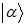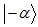##### High Density Optical Memories for Safe Archival Data

S. Kalbitzer

Physical Science International Journal, Page 366-371
DOI: 10.9734/PSIJ/2014/6711

Ion nanobeams are used to write data bits of nanometer diameter into storage materials and photon near-field technology is employed to read this novel kind of digital memory.

##### Improving the Teleportation of Superposition of Entangled Coherent States

Shivani A. Kumar

Physical Science International Journal, Page 339-344
DOI: 10.9734/PSIJ/2014/6307

I consider the teleportation of superposition of coherent statesandusing beam splitters and phase shifters. I propose some modifications which consist of writing the output state as a superposition of the vacuum state and nonzero even photon state, which allow for an almost perfect teleportation to be achieved for an appreciable mean number of photons.

##### The Weighed Average Geodetic of Distributions of Probabilities in the Statistical Physics

V. V. Ryazanov

Physical Science International Journal, Page 345-354
DOI: 10.9734/PSIJ/2014/3910

The results obtained in [(1; 2)] for the statistical distributions at studying algebra of decision rules and natural geometry generated by it, are applied to estimations of the nonequilibrium statistical operator and superstatistics. The expressions for the nonequilibrium statistical operator and superstatistics are derived as special cases of the weighted geodetic average of the probability distributions.

##### Studying the Effect of Vertical Eddy Diffusivity on the Solution of Diffusion Equation

Khaled S. M. Essa

Physical Science International Journal, Page 355-365
DOI: 10.9734/PSIJ/2014/5866

The advection diffusion equation (ADE) is solved in two directions to obtain the crosswind integrated concentration. The solution we used Laplace transformation technique and considering the wind speed depends on the vertical height and eddy diffusivity depends on downwind and vertical distances. The two predicted concentrations and observed concentration data taken on the Copenhagen in Denmark were compared.

##### Improvement in Gasochromic Properties of Tungsten Trioxide Using Optimized Pd Doping

M. Ameri, A. Nikfarjam, K. A. Rahmanzade

Physical Science International Journal, Page 372-383
DOI: 10.9734/PSIJ/2014/5663

Tungsten oxide layers are important structures in gasochromic and optical hydrogen sensing devices. In the present study, these layers were constructed using the sol-gel method and spin-coating deposition technique.  layers were coated with palladium using the same method to investigate the catalytic role of palladium on the surface of the layers. This catalyst was used to coat the  layers in the form palladium chloride using different concentrations of palladium. The layers were heated at different temperatures to study the gasochromic properties of the  layer in different crystalline and amorphous phases. Scanning electron microscopy (SEM), transmission electron microscopy (TEM), x-ray diffraction (XRD) and spectrophotometer analysis were used to investigate the surface morphology, structure of layers and size of the nanoparticles. When the colored glass was exposed to air, the sensor returned to its initial transmission state. The results of XRD spectral analysis showed that the layers commenced crystallization at 300°C and were completely crystallized at 500°C. The effects of temperature and catalyst concentration are investigated simultaneously. Optimal conditions were achieved at 300°C and for the 0.05M concentration of palladium chloride solution. Under this condition, after applying 2.7% hydrogen gas, the transmission change of the sample (ΔT1%=Ta-Tb , where Ta is initial transmission and Tb is coloring state of glass) was 47% and the response time was 3s. The transmission change (ΔT2%=Tc-Tb , where Tc is bleaching and Tb is coloring state of glass) and recovery time were 22% and 25s, respectively, after exposure of the sensor to air.

##### Geometric Phase, Curvature and the Monodromy Group

Bernard H. Lavenda

Physical Science International Journal, Page 384-401
DOI: 10.9734/PSIJ/2014/6837

The aim of this study is to show that geometric phase is a consequence of curvature in non-Euclidean geometries being related to the areas of spherical and hyperbolic triangles. In hyperbolic geometry it is well-known that the angular deficit of a hyperbolic triangle is related to Wigner rotation and Thomas precession, whereas in spherical geometry, its relation to automorphic functions arising from Fuchsian differential equations containing non-essential singularities has not been appreciated. It is the aim of this paper to fill this lacuna. Fuchsian differential equations with non-essential singularities are solved by a power series solution (indicial equation) and the quotient of two solutions will undergo linear-fraction transformations which tessellate the half-plane or unit disc with curvilinear triangles or lunes depending on the number of singular points. Their inverse is multivalued, periodic or automorphic, functions.  Analytic continuation   about a singular point does not give back the original solution. Multivaluedness is the cause of geometric phase. Examples are the Pancharatnam phase of beams of polarized light, the Aharonov-Bohm effect, the Dirac monopole and angular momenta with ‘centripetal’ attraction in the case of spherical geometry. These will be compared with non-collinear Lorentzian boosts that are responsible for Wigner rotation and Thomas precession in hyperbolic geometry, where the angle defect is related to the Euclidean measure of hyperbolic distance of two sides of a hyperbolic triangle in velocity space. For a right hyperbolic triangle, the angular defect is the angle of parallelism. A finite geometric phase requires non-integral quantum numbers and thus cannot be associated with ‘particles’. By conformal transformation, the homologues of the poles can be transformed into vertices of lunes, curvilinear triangles and polygons which place restrictions on the range of angular momenta. In contrast to quantum mechanics, where space is continuous and quantum numbers discrete, the space is now discrete, made up of tessellations which are repetitions of the fundamental region without lacunae and without overlap and the interval of the quantum numbers is continuous. Many of the equations of mathematic physics can be reduced to second-order Fuchsian equations with real coefficients in the limit of vanishing kinetic energy where essential singularities are reduced to simple poles. For only then will the solutions to the differential equations be rational functions in order that the covering group will be cyclic and the covering space will be a ‘spiral staircase’ like the different leaves of a Riemann surface.

##### Introducing Some Correlations to Calculate Entropy Generation in Extended Surfaces with Uniform Cross Sectional Area

Masoud Asadi, Amir Shalchi-Tabrizi

Physical Science International Journal, Page 402-415
DOI: 10.9734/PSIJ/2014/5157

The optimum length of extended surfaces with uniform cross sectional area has been analyzed numerically, based on the concept of entropy generation minimization. The extended surface studied is a pin fin. The rate of entropy generation is investigated for different boundary conditions. First, some correlations are introduced to calculate this rate, and then a model is offered to find optimum length of the fin for adiabatic and convection heat transfer boundary conditions. The accuracy of the model presented is compared with experimental data. Although Bejan introduced a correlation to calculate optimal Reynolds number and consequently the optimum length of a pin fin, but the results showed the new method has high accuracy compared with the Bejan method. Also, it is found that there is a strong relation between optimum length (based on the entropy generation minimization concept) in one side, and temperature distribution in the other side.

##### Measurements of Absolute Atomic Oxygen Density by Two-photon Absorption Laser-induced Fluorescence Spectroscopy in Hot Air Plasma Generated by Microwave Resonant Cavity

F. Marchal, M. Yousfi, N. Merbahi, A. Piquemal

Physical Science International Journal, Page 416-433
DOI: 10.9734/PSIJ/2014/6986

Hot air plasma is generated inside a 2.45GHz microwave resonant cavity and is ejected towards a gas conditioning cell at 600 mbar. A flow of 12 liter/min of dry air with a small amount of H2, in order to better detect OH (A-X) spectra for gas temperature measurements, is injected at the entrance of the resonant cavity using an input power of 1 kW. The measurement of absolute density of atomic ground state oxygen is determined using two-photon absorption laser-induced fluorescence (TALIF) spectroscopy for several radial and axial positions of the hot air plasma column which is a post discharge propagating inside the cell. The gas temperature corresponding to the radial and axial positions, where atomic oxygen density is measured, varies between about 3500K in the column axis down to about 2500K in the plasma border. The measured absolute oxygen density using xenon for calibration varies between about 2.1x1017cm-3 in the axis down to 0.05x1017cm-3. This leads to a dissociation degree of oxygen inside the post discharge air plasma column lower than the case where only local thermodynamic equilibrium is assumed. This deviation is due to non-thermal equilibrium effects that can be associated to the not yet achieved relaxation of the long-lived excited species during such post discharge stage.

##### Focusing of Optical Vector-vortex Beams

Geo M. Philip, Nirmal K. Viswanathan

Physical Science International Journal, Page 434-446
DOI: 10.9734/PSIJ/2014/6718

Theoretical formalism using vectorial Rayleigh diffraction integrals is developed to calculate the electric field components Ex, Ey, Ez of generalized vector-vortex (VV) beams of different phase and polarization characteristics as a function of propagation distance ‘z’ in the focal region of an axicon. This formalism is used to generate sub-wavelength spot-size (0.43λ) ultra-long length (80λ) longitudinally-polarized optical needle beam by appropriately selecting the phase and polarization characteristics of the input VV beam. The formalism is further extended to also generate purely transverse polarized beam with similar characteristics. The focusing process leads to interference between different field components of the beam resulting in the formation of C-point polarization singularities of index Ic = ±1 whose transverse characteristics evolve with propagation distance. Experimental results to support our theoretical calculations are presented along with lens focus comparison results.

##### A Review on Condition Monitoring and Diagnostic Techniques of Rotating Electrical Machines

S. A. Mortazavizadeh, S. M. G. Mousavi

Physical Science International Journal, Page 310-338
DOI: 10.9734/PSIJ/2014/4837

Electrical machines are critical components in industrial processes. A motor failure may yield an unexpected interruption at the industrial plant, with consequences in costs, product quality, and safety. To determine the conditions of each part of motor, various testing and monitoring methods have been developed. In this paper, a review on effective fault indicators and condition monitoring methods of rotating electrical machines has been accomplished. Fault detection methods divided to four groups: electrical, mechanical, chemical and thermal indicators. Some fault detection methods based on electrical symptoms like stator current, voltage, their combination or spectrum discussed in electrical group. In second branch, mechanical symptoms like torque, vibration and so on used for condition monitoring. Third group, chemical indicators, assigned to some chemical parameters of materials like oil characteristic or wear and debris in oil analysis. In last group, thermal symptoms in rotating electrical machines will be spoken. Between all methods, some of them are more known like vibration and some of them are recently added like motor current signature analysis (MCSA). Nowadays, combined methods and methods used artificial intelligence (AI) in condition monitoring are more popular. In every group, the fault detection method and the faults that can be detected have been mentioned. Mathematical equations of some new signal processing method have been discussed in literature presented in appendix.